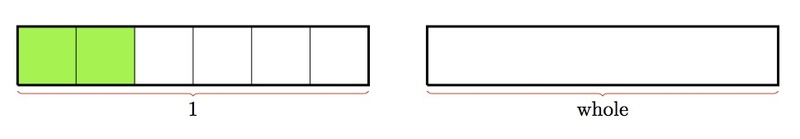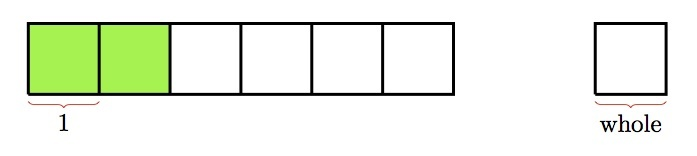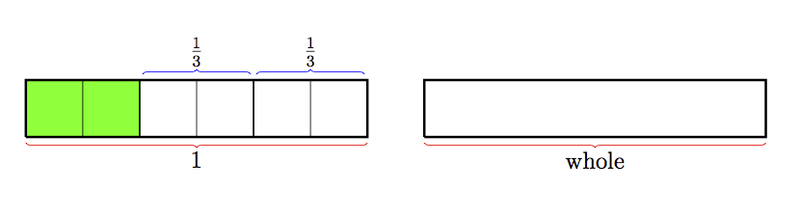# Naming the Whole for a Fraction

Alignments to Content Standards: 3.NF.A.1

Mrs. Frances drew a picture on the board.Then she asked her students what fraction it represents.

• Emily said that the picture represents $\frac{2}{6}$. Label the picture to show how Emily's answer can be correct.
• Raj said that the picture represents $\frac{2}{3}$. Label the picture to show how Raj's answer can be correct.
• Alejandra said that the picture represents 2. Label the picture to show how Alejandra's answer can be correct.

## IM Commentary

When fractions are represented pictorially, they are always fractions of some whole. It is sometimes easy to forget to indicate this in a picture, particularly because the idea may be clear in our own mind. The goal of this task is to show that when the whole is not specified, which fraction is being represented is left ambiguous. The teacher may wish to simply draw this picture on the board and have students think about what fraction the picture represents and follow this up with a discussion. When the whole is not specified for a fraction, a picture can represent an infinite number of different fractions: in this case there could be any number of small boxes (1,2,3, ...) in a whole and each possibility leads to a different meaning for the shaded or unshaded part of the picture.

The Standards for Mathematical Practice focus on the nature of the learning experiences by attending to the thinking processes and habits of mind that students need to develop in order to attain a deep and flexible understanding of mathematics. Certain tasks lend themselves to the demonstration of specific practices by students. The practices that are observable during exploration of a task depend on how instruction unfolds in the classroom. While it is possible that tasks may be connected to several practices, only one practice connection will be discussed in depth. Possible secondary practice connections may be discussed but not in the same degree of detail.

This particular task supports the demonstration of Mathematical Practice Standard 6, Attend to precision. Students are faced with elements of ambiguity in deciding what encompasses the whole. The whole in turn impacts the representation of a specific fraction. Students learn to appreciate, understand, and use mathematical vocabulary more precisely in interpreting and describing the whole and its fractional parts. While using different representations, such as pictures, they use appropriate labels to communicate the meaning of their representation (in this task the whole fraction and its accompanying fractional part).  Students also understand the possibility of different interpretations and therefore the necessity for precision in their work.

## Solutions

Solution: Interpreting the fractions as representing the shaded portion

• Emily's answer of $\frac{2}{6}$ would mean that all six squares together, make up the whole and two of these have been shaded:• For Raj, there are $3$ squares that make a whole so the two shaded squares represent two out of three or $\frac{2}{3}$:• For Alejandra, each square represents one whole as pictured below:Note that in addition to specifying what represents the whole in the picture, each whole has been outlined with thicker lines: this is a second way of indicating and reinforcing the meaning of the whole in the picture. Also, the whole itself is not a number so we have to be careful to clarify what part of the picture represents a fraction of the given whole.

Solution: Interpreting the fractions as representing the unshaded portion

For Raj, the unshaded part of the picture can naturally be interpreted to represent $\frac{2}{3}$. For this, the whole would be composed of all 6 squares as is indicated in the picture below:Then 2 squares represents $\frac13$ and the unshaded portion represents $\frac23$.

For Alejandra, too, the unshaded portion can be interpreted as representing 2 if a pair of squares represents the whole. This is pictured below: